Libri di Mohd T. Alodat - libri Statistica & Applicazioni

# Mohd T. Alodat

### Author's titles

Generalized Alpha Skew Normal Distribution digital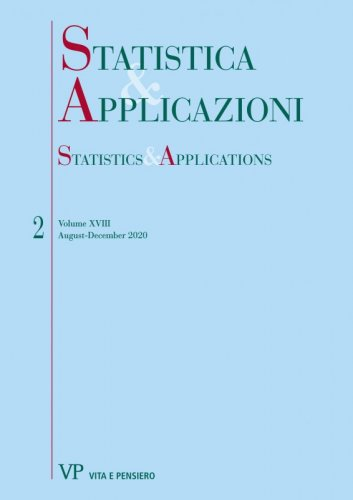Year: 2020
This paper extends the work of Elal-Olivero (2010) on the alpha-skew normal distribution. The extension is a multivariate version of Elal-Olivero’s univariate case. Then we study the statistical properties of the new extension such as marginal and conditional distribution, closure under convolution with normal random variate. Furthermore, we illustrate the performance of the distribution using simulated data obtained from the generalized distribution via the Metropolis-Hasting algorithm...
Increasing the efficiency of ranked set sampling via visual grouping with respect to a threshold digital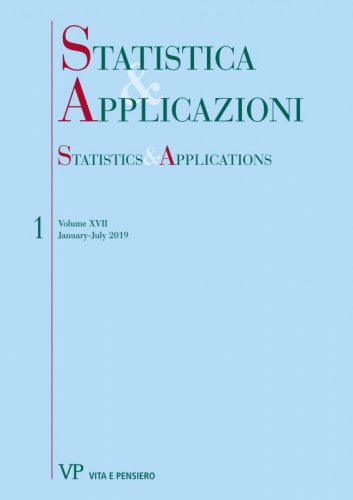Year: 2019
In this paper, a new sampling technique is proposed that carries more information than contained in ranked set sample (RSS). The proposed sampling technique is defined by making the use for the idea of visual grouping of population units with respect to a fixed threshold and RSS. We refer to it as RSS-Grouping technique. Under the best informative RSS-Grouping technique, the maximum likelihood estimator (MLE) of the mean of an exponential distribution is derived...
A Generalized multivariate skew-normal distribution with applications to spatial and regression predictions digital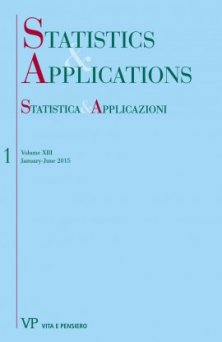Year: 2015
In this paper, a generalization to the multivariate skew-normal distribution of Arnold and Beaver (2002) is proposed. Also several distributional properties of the proposed distribution are explored. The proposed distribution has been used to define a stochastic process called the generalized-skew Gaussian process...
Estimating the normal population with known coefficient of variation using the optimal ranked set sampling scheme
Free
digital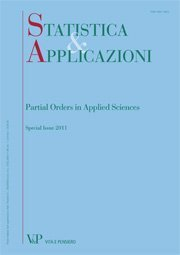Year: 2012
SUMMARY In the ranked set sampling with set size equal to m, there are mm different possible sampling schemes. The aim of this paper is to characterize the optimal sampling scheme via the Fisher information numbers for normal distribution with known coefficient of variation. Moreover, we use the optimal sampling schemes to obtain unbiased estimators for the mean. Also we compare the estimators to their counterparts under the McIntyre’s ranked set sampling scheme. We show that the MLE under optimal sampling scheme is asymptotically more efficient than its counterpart under McIntyre’s RSS. Keywords: Ranked Set Sampling, Fisher Information, Maximum Likelihood, Normal Distribution, Coefficient of Variation.
Chi-Square goodness of fit test for logistic distribution using RSS and SRS
Free
digitalYear: 2011
In this paper, we introduce a new method to improve the power of the chi-square test for goodness of fit (GOF) of logistic distribution under selective order ranked Set sampling (RSS) based on minimum, median and maximum as compared to simple random sampling (SRS). Based on a simulation study, we illustrate that the chi-square test is found to be more powerful under selective order RSS when compared to SRS, particularly for minimum and maximum. However, for the case of median RSS, the test is found to be more powerful under SRS. To investigate these results further, the Kullback- Leibler information (KLI) is applied and similar results are found. Keywords: Goodness of fit test, chi-square test, logistic distribution, ranked set sample, simple random sample.
Median ranked set sampling for polynomial regression
Free
digital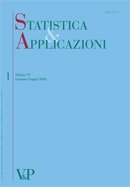Year: 2011
SUMMARY The purpose of this article is to study the ploynomial linear regression model under the median ranked set sampling (MRSS) scheme introduced by Muttlak (1997). If the response variable can be more easily ranked than quantified, then we use the MRSS to collect data by ranking on the response variable. We obtain estimators and confidence intervals for the polynomial regression parameters under MRSS when the errors have a symmetric distribution. We also show that the least square estimators, under MRSS, are more efficient than their SRS counterparts and give illustrating examples. Keywords: Median Ranked Set Sample, Polynomial Regression.
Comparison of several confidence intervals for normal distribution with known coefficient of variation
Free
digitalYear: 2010
In this paper, we consider the problem of constructing confidence intervals for the normal population mean when the coefficient of variation is known. We obtain 13 confidence intervals using different pivots. Also we conduct a simulation study to compare the expectations as well as the standard deviations of the intervals lengths. Keywords: Coefficient of Variation, Normal Distribution, Pivotal Method, Confidence Interval.
Moving extreme ranked set sampling for simple linear regression
Free
digitalYear: 2009
The moving extreme ranked set sampling, introduced by Alodat and Al-Saleh (2001), is a modification of the well known ranked set sampling approach that was proposed by McIntyre (1952). In this paper, we suggest new estimators for the simple linear regression parameters under the moving extreme ranked set sampling scheme. Moreover, we show that the proposed estimators are more efficient than their counterparts using the simple random sampling approach. We illustrate our ideas and thoughts via simulation and data analysis and conduct a comparison between our approach and the traditional ones. Keywords: Moving ranked set sampling, Ranked set sampling, Simple linear regression.
Estimation of power function distribution with application to ecological relative abundance
Free
digitalYear: 2008
In this paper we derive Bayesian and non-Bayesian estimators for the parameter of the power function distribution, and prediction intervals for the maximum of a future sample. We apply our approach to field data of plant species relative abundance, the abundance of a given species divided by the total abundance of all plant species in given a community, collected in a biodiversity project in central Europe.